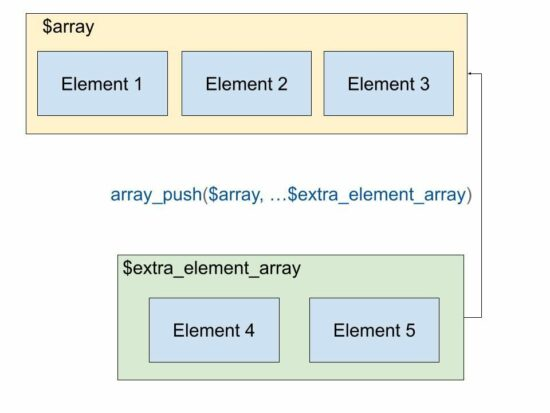# PHP array_push – Add Elements to an Array

Adding elements to an array in PHP is very easy with its native function array_push().

This quick example shows the simplicity of this function to add more elements to the end of an array.

## Quick example

``````<?php
\$animalsArray = array(
"Lion",
"Tiger"
);
array_push(\$animalsArray, "Elephant", "Horse");
print_r(\$animalsArray);
?>
``````

Output:

``````Array (  => Lion  => Tiger  => Elephant  => Horse )
``````

PHP array_push() function add elements to an array. It can add one or more trailing elements to an existing array.

Syntax

``````array_push(array &\$array, mixed ...\$values): int
``````
• \$array – The reference of a target array to push elements.
• \$values – one or more elements to be pushed to the target array.

When we see the PHP array functions, we have seen a short description of this function.## All possible ways of doing array push in PHP

In this tutorial, we will see all the possibilities for adding elements to an array in PHP. Those are,

• Array push by assigning values to an array variable by key.
• Pushing array elements in a loop.

When seeing the examples, it will be very simple and may be too familiar also. But recollecting all the methods at one glance will help to rejuvenate the skillset on basics.

## How to add an array of elements to a target array using array_push()

This example uses the PHP array_push() function to push an array of elements into a target array.

``````<?php
\$animalsArray = array(
"Lion",
"Tiger"
);
\$anotherArray = array(
"Elephant",
"Crocodile"
);

array_push(\$animalsArray, ...\$anotherArray);
print_r(\$animalsArray);
// this method adds elements from two arrays sequentially into the target array
// this is similar to merge
?>
``````

Output:

``````Array (  => Lion  => Tiger  => Elephant  => Crocodile )
``````

## The alternate method to array_push

The array_push function is useful if there is a requirement to push elements later after the alignment.

If you want to push the elements at an assignment level the following code shows the way to do it.

If you want to merge JSON array or object using PHP the linked article will be helpful.

``````<?php
// alternate to use array_push when you have a key value
// add elements as key value via index
\$animalsArray['a1'] = 'Lion';
\$animalsArray['a2'] = 'Tiger';
\$animalsArray['a3'] = 'Elephant';
\$animalsArray['a4'] = 'Horse';
print_r(\$animalsArray);
?>
``````

Output:

``````Array ( [a1] => Lion [a2] => Tiger [a3] => Elephant [a4] => Horse )
``````

## Pushing elements into an array in a loop without using array_push

This code is the same as above but with a PHP for a loop. It pushes only the value to the array variable with a square bracket.

The output will have the array with a numerical key.

``````<?php
// another alternate to array_push
// add elements to an array with just []
\$array = array();
for (\$i = 1; \$i <= 10; \$i ++) {
\$array[] = \$i;
}
print_r(\$array);
?>
``````

If you want to push the key-value pair to form an associative array with a loop, the following code will be helpful.

Output:

``````Array (  => 1  => 2  => 3  => 4  => 5  => 6  => 7  => 8  => 9  => 10 )
``````

## Adding elements into an array using PHP array_merge()

The array_merge() and the array_push(\$array, …\$array_sequence) gives same output.

It merges two array variables and results in a consolidated element array. If you want to merge JSON array or object in PHP the linked article has the code.

``````<?php
\$animalsArray = array(
"Lion",
"Tiger"
);
\$moreAnimalsArray = array(
"Elephant",
"Horse"
);
// to add elements in an array from existing arrays
\$array = array_merge(\$animalsArray, \$moreAnimalsArray);
print_r(\$array);
?>
``````

Output:

``````Array (  => Lion  => Tiger  => Elephant  => Horse )
``````

## PHP function to add elements to the beginning of an array

PHP also contains functions to add elements to an array at the beginning of an array. The array_unshift() function is used for this. See the following code that adds elements to an array using array_shift().

``````<?php
\$animalsArray = array(
"Lion",
"Tiger"
);
array_unshift(\$animalsArray, "Elephant", "Horse");
print_r(\$animalsArray);
?>
``````

Output:

``````Array (  => Elephant  => Horse  => Lion  => Tiger )
``````